## 一篇文章带你了解SVG 转换知识

SVG 转换在SVG图像中创建的形状。例如，移动，缩放和旋转形状。这是显示垂直或对角线文本的便捷方法。

# 一、转换简单示例

``````<svg xmlns="http://www.w3.org/2000/svg"

<rect x="50" y="50" height="110" width="110"
style="stroke:#ff0000; fill: #ccccff" transform="translate(30) rotate(45 50 50)">
</rect>
<text x="70" y="100" transform="translate(30) rotate(45 50 50)"
>nhooo.com</text>
</svg>
``````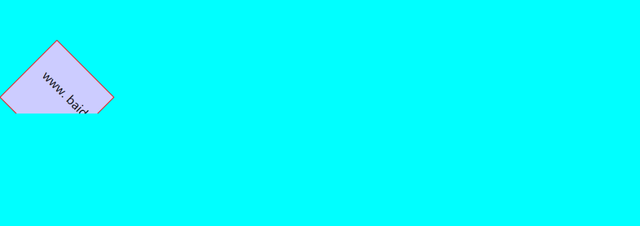# 1. 移动 translate()

translate()函数移动形状。将x 和 y 值传递给translate()的函数。

``````translate(50,25)
``````

``````<svg width="500" height="150">
<rect x="20" y="20" width="50" height="50" style="fill: #cc3333" />
<rect x="20" y="20" width="50" height="50" style="fill: #3333cc" transform="translate(75,25)">
</rect>
</svg>
``````# 2. 旋转 rotate()

rotate()函数围绕点0,0旋转形状。

``````<svg width="500" height="150">
<rect x="20" y="20" width="40" height="40" style="stroke: #3333cc; fill:none;"></rect>

<rect x="20" y="20" width="40" height="40" style="fill: #3333cc" transform="rotate(15)"></rect>
</svg>
``````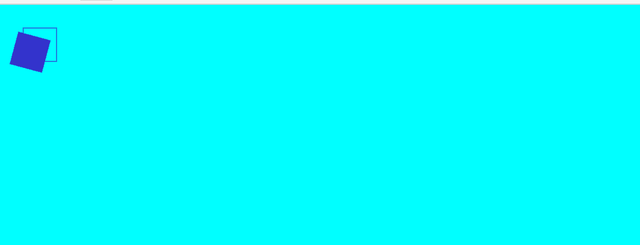``````<svg width="500" height="150">
<rect x="20" y="20" width="40" height="40"
style="stroke: #3333cc; fill:none;"
></rect>

<rect x="20" y="20" width="40" height="40"
style="fill: #3333cc"
transform="rotate(15, 40, 40)"
></rect>
</svg>
``````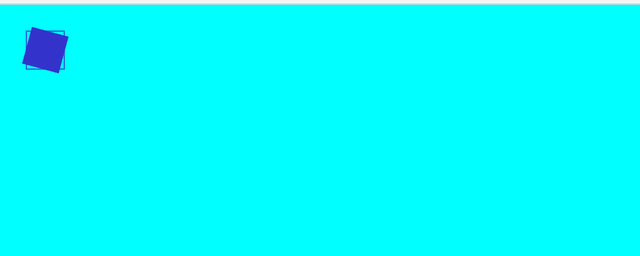# 3.1 scale()函数简介

scale()函数按比例放大或缩小形状。scale()函数可缩放形状尺寸及其位置坐标。因此，以20乘以2的比例缩放的宽度为20且高度为30的矩形位于20,20处，其宽度为40且高度为60。

scale()函数还可以缩放形状的笔触宽度。

# 3.2 案例

``````<svg width="500" height="150">
<rect x="10" y="10" width="20" height="30" style="stroke: #3333cc; fill:none;"></rect>

<rect x="10" y="10" width="20" height="30" style="stroke: #000000; fill:none;" transform="scale(2)"></rect>
</svg>
``````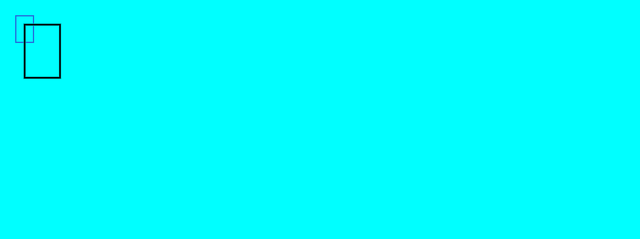``````scale(2,3);
``````

``````<svg width="500" height="150">
<rect x="10" y="10" width="20" height="30" style="stroke: #3333cc; fill:none;"></rect>

<rect x="10" y="10" width="20" height="30" style="stroke: #000000; fill:none;" transform="scale(2,3)"></rect>
</svg>
``````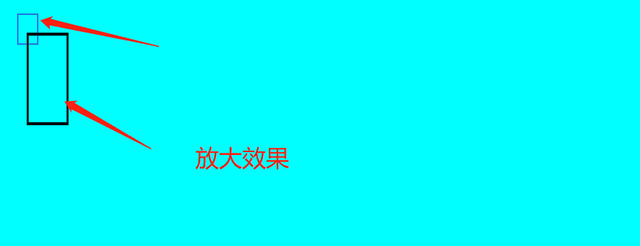# 4. 偏斜 skew()

skewX()和skewY()函数偏斜x轴和y轴。实际上，这些函数会根据以度为单位指定的某个角度来倾斜给定的轴。

``````<svg width="500" height="150">
<rect x="10" y="10" width="20" height="30" style="stroke: #3333cc; fill:none;" />

<rect x="50" y="10" width="20" height="30" style="stroke: #000000; fill:none;" transform="skewX(10)" />
<rect x="100" y="10" width="20" height="30" style="stroke: #000000; fill:none;" transform="skewX(45)" />
<rect x="150" y="10" width="20" height="30" style="stroke: #000000; fill:none;" transform="skewX(60)" />
</svg>
``````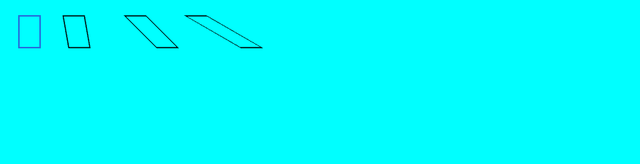skewX()函数使垂直线看起来像是按给定角度旋转了。

``````<svg width="500" height="150">
<rect x="10" y="10" width="20" height="30" style="stroke: #3333cc; fill:none;" />

<rect x="50" y="10" width="20" height="30" style="stroke: #000000; fill:none;" transform="skewY(60)" />
<rect x="100" y="10" width="20" height="30" style="stroke: #000000; fill:none;" transform="skewY(45)" />
<rect x="150" y="10" width="20" height="30" style="stroke: #000000; fill:none;" transform="skewY(10)" />
</svg>
``````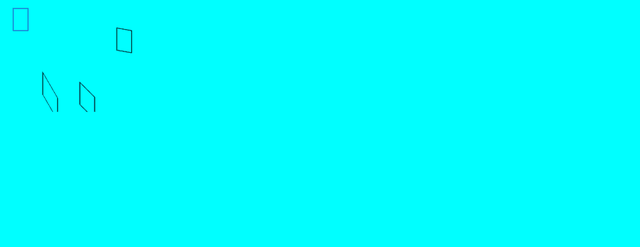# 四、总结

IT共享之家——————- End ——————